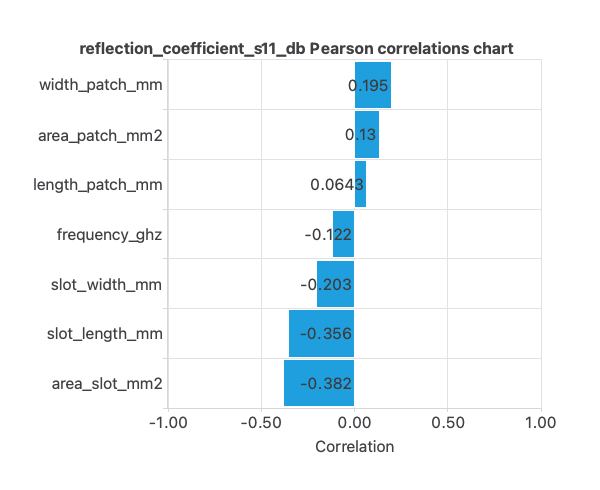A patch antenna is a wireless antenna that uses a flat, rectangular metal patch on a substrate to transmit or receive radio frequency (RF) signals. The coefficient of reflection, S11, measures the power reflected to the source when RF signals are transmitted through the patch antenna. S11 is typically expressed as a ratio of power, with values close to 0 indicating tiny reflection and values close to 1 indicating significant reflection. This example evaluates the reflection coefficient of patch antennas with different features. We used data from 8 antennas, including variables extracted from their geometries.

### Contents

This dataset was generated using HFSS software, and the radiation frequency was kept at 2.4 GHz, commonly used for Bluetooth and WLAN operations, and can be used to optimize the design and performance of patch antennas.

## 1. Application type

The predicted variable in this application is the coefficient of reflection, S11, for a patch antenna. Therefore, this is an approximation project.

The goal is to optimize the antenna design to obtain the lowest possible value for S11, which indicates a high level of power being transmitted and a low level of reflection using artificial intelligence and machine learning.

## 2. Data set

This dataset features information on patch antennas, a type of microstrip antenna widely used in wireless communications. The dataset includes various dimensions and parameters of the antenna, including the operating frequency, dimensions of the patch and slots, and a measure of the antenna’s reflection coefficient. The data is organized in clusters; there are 8 clusters in total.

The number of input variables, or attributes for each sample, is 7. The target variable is S11. The following list summarizes the variables information:

• frequency_ghz: The operating frequency of the antenna, measured in gigahertz.
• length_patch_mm: The length of the patch antenna, measured in millimeters.
• width_patch_mm: The width of the patch antenna, measured in millimeters.
• area_patch_mm2: The total area of the patch antenna, measured in square millimeters.
• slot_length_mm: The length of the slots on the antenna, measured in millimeters.
• slot_width_mm: The width of the slots on the antenna, measured in millimeters.
• area_slot_mm2: The total area of the slots on the antenna, measured in square millimeters.
• reflection_coefficient_s11_db: The S11 parameter, measured in decibels, measures the antenna’s reflection coefficient.

To start, we use all instances. Each instance contains the input and target variables of a different patient. The data set is divided into training, validation, and testing subsets. Neural Designer automatically assigns 60% of the instances for training, 20% for selection, and 20% for testing.

The inputs-targets correlations might indicate to us which factors have the most univariate influence on whether or not a patient will live.Here, the most correlated variables with survival status are area_slot_mm2 and slot_length_mm.

## 3. Neural network

The next step is to set a neural network representing the approximation function.
For this class of applications, the neural network is composed of:

The scaling layer contains the statistics on the inputs calculated from the data file and the method for scaling the input variables. Here, the minimum-maximum method has been set. As we use 7 input variables, the scaling layer has 7 inputs.

We use 2 perceptron layers here:

The unscaling layer contains the statistics of the output.

The following figure is a graphical representation of this neural network.## 4. Training strategy

The fourth step is to set the training strategy, which is composed of two terms:

• A loss index.
• An optimization algorithm.

The loss index is the weighted squared error with L2 regularization, which is the default loss index for binary classification applications.

We can state the learning problem as finding a neural network that minimizes the loss index.
That is a neural network that fits the data set (error term) and does not oscillate (regularization term).

The optimization algorithm that we use is the quasi-Newton method which is also the standard optimization algorithm for this type of problem.

The following chart shows how the error decreases with the iterations during the training process.
The final training and selection errors are training error = 0.153 WSE and selection error = 0.205 WSE, respectively.As we can see in the previous image, the curves have converged.

## 5. Testing analysis

The objective of the testing analysis is to validate the performance of the generalization properties of the trained neural network. In this case, we show the minimums, maximums, mean and standard deviations of the absolute and percent errors of the neural network for the testing data.As we can see, the average error is around 1%.

Additionally, the erros histogram shows the distribution of the erros from the neural network on the testing samples. In general, we expect a normal distribution like this.As we can observe, most of the errors are around 0.

## 6. Model deployment

Once we have tested the neural network’s generalization performance, we can save it for future use in the so-called model deployment mode.

The mathematical expression represented by the neural network is written below.

scaled_frequency_ghz = (frequency_ghz-2.408770084)/0.6040109992;
scaled_length_patch_mm = (length_patch_mm-39.63669968)/20.70210075;
scaled_width_patch_mm = (width_patch_mm-50.44079971)/34.84690094;
scaled_area_patch_mm2 = (area_patch_mm2-2701.02002)/3742.169922;
scaled_slot_length_mm = (slot_length_mm-19.39929962)/11.01990032;
scaled_slot_width_mm = (slot_width_mm-26.85779953)/16.36989975;
scaled_area_slot_mm2 = (area_slot_mm2-671.4219971)/1431.26001;

perceptron_layer_1_output_0 = tanh( -6.33168 + (scaled_frequency_ghz*1.30197) + (scaled_length_patch_mm*1.41481) + (scaled_width_patch_mm*-2.04832) + (scaled_area_patch_mm2*0.646831) + (scaled_slot_length_mm*2.73) + (scaled_slot_width_mm*-1.89205) + (scaled_area_slot_mm2*0.0614876) );
perceptron_layer_1_output_1 = tanh( -2.28184 + (scaled_frequency_ghz*5.21126) + (scaled_length_patch_mm*0.803737) + (scaled_width_patch_mm*0.268686) + (scaled_area_patch_mm2*-0.136691) + (scaled_slot_length_mm*-0.161648) + (scaled_slot_width_mm*-2.42782) + (scaled_area_slot_mm2*2.42202) );
perceptron_layer_1_output_2 = tanh( 2.32082 + (scaled_frequency_ghz*-5.54642) + (scaled_length_patch_mm*-3.07181) + (scaled_width_patch_mm*1.70944) + (scaled_area_patch_mm2*0.455758) + (scaled_slot_length_mm*0.289671) + (scaled_slot_width_mm*2.28278) + (scaled_area_slot_mm2*-1.07915) );

perceptron_layer_2_output_0 = ( -5.7008 + (perceptron_layer_1_output_0*-5.91444) + (perceptron_layer_1_output_1*4.95589) + (perceptron_layer_1_output_2*5.0324) );

unscaling_layer_output_0=perceptron_layer_2_output_0*3.042239904-1.718469977;